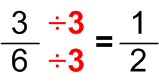# Simplifying numerical fractions

Simplifying numerical fractions is a very usefull skill and knowing how to make fractions simpler can make a world of diference. If you are solving an equation in which there are fractions with large numerators or denominators, reducing those fractions to their lowest value will make finding the result much easier.  In order to make a fraction simpler, the fraction needs to have at least two numbers that could be divided with same number. For example; you have a fraction 20/40 that can be simplified because both numerator and denominator could be divided with same number (in this case, it is number 20). In the end, you will get the number 1/2 as the result. Dividing both the numerator and the denominator with the same number is very important since it is the only way their ratio will not change and therefore influence the end result. If you want to simplify the fraction as much as possible, the number you use for simplifycation should be the greatest common factor of the numerator and the denominator.

For practice, you can use the worksheets below. They should provide you with enough practice material to help you master this skill as soon as possible.## Simplifying numerical fractions exams for teachers

 Exam Name File Size Downloads Upload date Proper fractions Simplifying proper fractions – easy 460.6 kB 3475 September 3, 2019 Simplifying proper fractions – medium 460.6 kB 2711 September 3, 2019 Simplifying proper fractions – hard 461.8 kB 2811 September 3, 2019 Mixed numbers Simplifying mixed numbers – easy 459.8 kB 3159 September 3, 2019 Simplifying mixed numbers – medium 460.1 kB 2372 September 3, 2019 Simplifying mixed numbers – hard 460.2 kB 2573 September 3, 2019 Simplifying improper fractions – easy 460.5 kB 2585 September 3, 2019 Improper fractions Simplifying improper fractions – medium 460.5 kB 2513 September 3, 2019 Simplifying improper fractions – hard 460.7 kB 2344 September 3, 2019 Simplifying improper fractions into mixed numbers – easy 460.7 kB 2254 September 3, 2019 Simplifying improper fractions into mixed numbers – medium 459.9 kB 2048 September 3, 2019 Simplifying improper fractions into mixed numbers – hard 460 kB 2532 September 3, 2019

## Simplifying numerical fractions worksheets for students

 Worksheet Name File Size Downloads Upload date Simplifying proper fractions 503.3 kB 2824 September 3, 2019 Simplifying mixed numbers 509.2 kB 2578 September 3, 2019 Simplifying improper fractions into mixed numbers 506.3 kB 2187 September 3, 2019 Simplifying improper fractions 509.5 kB 2237 September 3, 2019